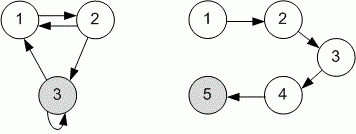Competitions

# Maximum by minimum

Directed unweighted graph is given. Find in it a vertex, the shortest distance from which to the given one is maximum, and print this distance.

#### Input

First line contains three positive integers n, m и s (1sn5000, 1m20000) - the number of vertices and edges in the graph and the number of given vertex. In the next m lines the graph edges are given. Each edge is given with the pair of numbers - the starting and the ending point.

#### Output

Print the required shortest distance.Time limit 2 seconds
Memory limit 128 MiB
Input example #1
3 5 3
1 2
2 1
3 1
2 3
3 3

Output example #1
2

Input example #2
5 4 5
1 2
2 3
3 4
4 5

Output example #2
4